•5星
1.24MB u013883025 2021-06-30 11:33:33
•5星
1KB weixin_42682754 2021-10-01 17:53:42
•5星
43KB qq_34093397 2021-01-04 10:45:38
•5星
2KB weixin_42696271 2021-09-11 06:14:32
•5星
1.05MB wlwdecs_dn 2021-01-20 08:52:45
•5星
61KB weixin_42666807 2021-09-29 06:06:38
•5星
39KB weixin_45317919 2021-06-04 11:27:28
•5星
178.06MB guoruibin123 2021-06-25 13:54:42
• (一)高斯低通滤波去噪高斯低通滤波器(Gaussian Low Pass Filter)是一...一维高斯函数和二维高斯函数 (高斯低通滤波器的传递函数) 的表达形式分别如下： 公式中，为标准差，由于图像通常是二维信号，因此图像去噪通常...

(一)高斯低通滤波去噪

高斯低通滤波器(Gaussian Low Pass Filter)是一类传递函数为高斯函数的线性平滑滤波器。又由于高斯函数是正态分布的密度函数。因此高斯低通滤波器对于去除服从正态分布(Normal distribution)的噪声非常有效。一维高斯函数和二维高斯函数 (高斯低通滤波器的传递函数) 的表达形式分别如下：公式中，为标准差，由于图像通常是二维信号，因此图像去噪通常使用二维高斯函数作为传递函数，而高斯函数具有可分离的特性，因此可以先对行进行高斯滤波，再对列进行高斯滤波，这样二维高斯函数就可以降为一维高斯滤波。下图1分别模拟了标准差为10和标准差为50的高斯函数。图1：不同标准差时的高斯曲线

从上图可以看出，高斯函数的标准差越大，高斯曲线越平滑。去噪能力越强，图像越模糊。

下图2用均值为0方差分别为0.1,0.5,1.0的高斯噪声对原图像进行污染的结果。图2

高斯滤波的实现方式有时域方式和频域方式两种，一种是时域高斯低通滤波，一种是频域高斯低通滤波。下面首先看看时域高斯低通滤波的结果。时域高斯低通滤波的实质是定义一个奇数大小的模板(3 X 3 ；5 X 5 ；7 X 7 ……)，然后让该模板遍历整副图像，模板中的加权平均值就是模板中心的值。时域高斯低通滤波的结果如下图所示：图3：不同的标准差和领域大小时的去噪后的图像

从上图可以看出，当领域窗口固定时，标准差越大，去除高斯噪声能力越强，图像越模糊，当标准差为2以上时，去噪能力几乎不再增加，只有当增加领域的大小时，去噪能力才会进一步增强。下面我们可以看一下，标准差分别为10和30的高斯曲线来进一步说明在邻域窗口大小一致的情况下，标准差越大，高斯曲线越宽，那么去高频噪声的能力就越强。但是他不是无限增强的，最终会趋于一个稳定值，只有当继续增大邻域窗口时，去噪能力才会进一步增强。图4：不同标准差时的高斯曲线

上述时域高斯低通滤波的matlab源代码如下：

%时域高斯低通去噪

subplot(231),imshow(x);title('高斯噪声为0.1的原图')

y1 = fspecial('gaussian',5,0.1);

z1 = imfilter(x,y1,'symmetric');

subplot(232),imshow(z1);title('领域窗口大小为5X5,标准差为0.1');

y2 = fspecial('gaussian',5,2);

z2 = imfilter(x,y2,'symmetric');

subplot(233),imshow(z2);title('领域窗口大小为5X5,标准差为2');

y3 = fspecial('gaussian',5,3);

z3 = imfilter(x,y3,'symmetric');

subplot(234),imshow(z3);title('领域窗口大小为5X5,标准差为3');

y4 = fspecial('gaussian',5,5);

z4 = imfilter(x,y4,'symmetric');

subplot(235),imshow(z4);title('领域窗口大小为5X5,标准差为5');

y4 = fspecial('gaussian',11,5);

z4 = imfilter(x,y4,'symmetric');

subplot(236),imshow(z4);title('领域窗口大小为11X11,标准差为5');

下面实现频域高斯低通滤波器

由于时域滤波的本质就是采用原始图像与滤波核(领域窗口)进行卷积的操作，我们知道卷积的运算速度是比较慢的，由傅里叶变换的性质可知，时域卷积可以转化为频域的乘积。因而频域高斯低通滤波应运而生。该部分内容基本源于冈萨雷斯版数字图像处理中第四章的内容，为了避免抄书，这里仅给出与时域滤波有相似结果的频域滤波的matlab源代码。

频域高斯低通滤波器的传递函数为：；Matlab自带了低通滤波器函数lpfilter；它的源代码如下：

function H = lpfilter(type, M, N, D0, n)

%LPFILTER Computes frequency domain lowpass filters.

% H = LPFILTER(TYPE, M, N, D0, n) creates the transfer function of

% a lowpass filter, H, of the specified TYPE and size (M-by-N). To

% view the filter as an image or mesh plot, it should be centered

% using H = fftshift(H).

%

% Valid values for TYPE, D0, and n are:

%

% 'ideal' Ideal lowpass filter with cutoff frequency D0. n need

% not be supplied. D0 must be positive.

%

% 'btw' Butterworth lowpass filter of order n, and cutoff

% D0. The default value for n is 1.0. D0 must be

% positive.

%

% 'gaussian' Gaussian lowpass filter with cutoff (standard

% deviation) D0. n need not be supplied. D0 must be

% positive.

[U, V] = dftuv(M, N);

% Compute the distances D(U, V).

D = sqrt(U.^2 + V.^2);

% Begin filter computations.

switch type

case 'ideal'

H = double(D <= D0);

case 'btw'

if nargin == 4

n = 1;

end

H = 1./(1 + (D./D0).^(2*n));

case 'gaussian'

H = exp(-(D.^2)./(2*(D0^2)));

otherwise

error('Unknown filter type.')

end

subplot(221)

imshow(f);title('原图')

f_r = f(:,:,1);

f_g = f(:,:,2);

f_b = f(:,:,3);

[M,N] = size(f_r);

sig1 = 50; %%截止频率

Fp_r = fft2(f_r,PQ(1),PQ(2)); %% R通道傅里叶变换

Fp_g = fft2(f_g,PQ(1),PQ(2));

Fp_b = fft2(f_b,PQ(1),PQ(2));

Hp1 = lpfilter('gaussian',PQ(1),PQ(2),2*sig1); %%生成高斯低通滤波，

Gp_r1 = Hp1.*Fp_r; %% R通道高斯低通滤波

Gp_g1 = Hp1.*Fp_g;

Gp_b1 = Hp1.*Fp_b;

gp_r1 = real(ifft2(Gp_r1)); %% 取得R通道的傅里叶反变换；

gp_g1 = real(ifft2(Gp_g1));

gp_b1 = real(ifft2(Gp_b1));

gpc_r1 = gp_r1(1:size(f,1),1:size(f,2));

gpc_g1 = gp_g1(1:size(f,1),1:size(f,2));

gpc_b1 = gp_b1(1:size(f,1),1:size(f,2));

gpc1 = cat(3,gpc_r1,gpc_g1,gpc_b1);

gpc1 = uint8(gpc1);

subplot(222),imshow(gpc1);title('截止频率为50的低通滤波')

%%下面计算截止频率为30的频域高斯低通滤波

sig2 = 30;

Hp2 = lpfilter('gaussian',PQ(1),PQ(2),2*sig2); %%生成高斯低通滤波，

Gp_r2 = Hp2.*Fp_r; %% R通道高斯低通滤波

Gp_g2 = Hp2.*Fp_g;

Gp_b2 = Hp2.*Fp_b;

gp_r2 = real(ifft2(Gp_r2)); %% 取得R通道的傅里叶反变换；

gp_g2 = real(ifft2(Gp_g2));

gp_b2 = real(ifft2(Gp_b2));

gpc_r2 = gp_r2(1:size(f,1),1:size(f,2));

gpc_g2 = gp_g2(1:size(f,1),1:size(f,2));

gpc_b2 = gp_b2(1:size(f,1),1:size(f,2));

gpc2 = cat(3,gpc_r2,gpc_g2,gpc_b2);

gpc2 = uint8(gpc2);

subplot(223),imshow(gpc2);title('截止频率为30的低通滤波')

%%下面计算截止频率为10的频域高斯低通滤波

sig2 = 10;

Hp3 = lpfilter('gaussian',PQ(1),PQ(2),2*sig2); %%生成高斯低通滤波，

Gp_r3 = Hp3.*Fp_r; %% R通道高斯低通滤波

Gp_g3 = Hp3.*Fp_g;

Gp_b3 = Hp3.*Fp_b;

gp_r3 = real(ifft2(Gp_r3)); %% 取得R通道的傅里叶反变换；

gp_g3 = real(ifft2(Gp_g3));

gp_b3 = real(ifft2(Gp_b3));

gpc_r3 = gp_r3(1:size(f,1),1:size(f,2));

gpc_g3 = gp_g3(1:size(f,1),1:size(f,2));

gpc_b3 = gp_b3(1:size(f,1),1:size(f,2));

gpc3 = cat(3,gpc_r3,gpc_g3,gpc_b3);

gpc3 = uint8(gpc3);

subplot(224),imshow(gpc3);title('截止频率为10的低通滤波')仿真结果如下：高斯低通滤波虽然较为简单，个人觉得将它说的非常明白，还是有些困难，这与自己的表达能力差有很大的关系，以后要养成些博客的习惯，希望能够尽早提高。我还是习惯用时域滤波的方法，频域滤波可以将时域的卷积运算转化为频域乘积运算，然而时域转化为频域过程中的傅里叶计算同样耗费时间。时域运算是领域操作，而频域计算式整体操作，关于时域和频域孰优孰劣还有待进一步考究。

高斯低通滤波应该是最基本的去噪手段，后面将进一步阐述双边滤波去噪、非局部均值去噪，以及核回归用于图像去噪。

-------------------------------------------任何一个理论，无论它是简单还是复杂，都应该将它剖析的非常清楚，这样才有可能将复杂的问题简单化，首先要将自己说服，才有可能让别人信服----------------------------------------------

展开全文weixin_39711441 2021-05-25 08:16:51
• Matlab对含噪声图像的滤波操作_两种噪声_三种滤波器_两种方法 matlab 卷积 信号处理

用到的两种方法： 直接conv2 fft2 %%C1 figure; tiledlayout(1,3); img = imread("\LenaG.bmp"); fft = fft2(img); nexttile; imshow(img); title("LenaG"); fft_shift = double(fftshift(fft)); fft_mag

注释很重要

Matlab对含噪声图像的滤波操作。

噪声：

• 高斯噪声（正态分布）
• 均匀噪声

用到的滤波器：

• 高斯滤波器
• 盒型滤波器
• 中值滤波器

用到的两种方法：

• 直接conv2
• fft2
%%C1
figure;
tiledlayout(1,3);
fft = fft2(img);
nexttile;
imshow(img);
title("LenaG");
fft_shift = double(fftshift(fft));
fft_magnitude_shift = abs(fft_shift);
nexttile;
imshow(log(fft_magnitude_shift),[]); %加对数以便于显示图像,为了更好地显示细节，不进行log变换的话灰度的动态范围被压缩
title("FFT with shift");
fft_angle_shift = angle(fft_shift);
nexttile;
imshow(fft_angle_shift,[]);%根据 C 中像素值的范围缩放显示.使用 [min（C（:)）max（C（:)）] 作为显示范围。 imshow 将 C 中的最小值显示为黑色，将最大值显示为白色。使用imshow(A,[])，即可把图像矩阵A显示为正常的灰度图像。本来A是0-1，把double拉伸到[0 255]
title("Phase with shift");

%%C2
figure;
tiledlayout(1,2);
dct = dct2(img);
nexttile
imshow(img);
title('LenaG');
dct_abs = abs(dct);
nexttile;
imshow(log(dct_abs), []);
%colormap(gca, gray)
colorbar;
title('Cosine transform');

%%C3
figure;
tiledlayout(1,2);
fft2_img = fft2(img);
hartley = real(fft2_img) - imag(fft2_img);
nexttile;
imshow(img);
title('LenaG')
hartley_abs = abs(hartley);
nexttile;
imshow(log(hartley_abs), []);
colormap(gca, gray);
colorbar;
title('Hatley');

%%C4 (a)
figure;
tiledlayout(1,2);
kernel_r = fspecial('gaussian', size(img), 10); % Compose Gaussian Kernel S.D. = 10
%两个函数，翻转其中一个，再滑动求积分，叫卷积（convultion）；不翻转就滑动求积分，叫做互相关（cross-correlation）。如果其中之一是偶函数，那么卷积和互相关效果相同。
%Two functions, flip one of them, and then slide for integration, called convultion; without flipping, slide for integration, called cross-correlation. If one of them is an even function, then convolution and cross-correlation have the same effect.
%卷积意味着把输入信号在时间轴上翻转，然后跟信号处理系统的描述方程（冲激响应）叠加积分.
%Convolution means flipping the input signal on the time axis, and then
%superimposing the integral with the description equation (impulse response) of the signal processing system.
kernel_r = rot90(kernel_r,2);
gaussian_img = img + uint8(20*randn(size(img)));
img = im2double(gaussian_img);%图片都以uint8数据储存。当对图片进行数据运算时，数据大于256就出现溢出，结果失真。而双精度数运算时不会出现溢出
nexttile;
imshow(img);
title("LenaG");
conv_img = conv2(img,kernel_r);
nexttile;
imshow(conv_img);
title("conv2 10");

%%C4 (all)
standard_deviation = [10, 20, 30, 5, 3];
kernels = {};
for i = 1:length(standard_deviation)
kernels{i} = fspecial('gaussian', size(img), standard_deviation(i)); % Compose Gaussian Kernel S.D. = 10
%两个函数，翻转其中一个，再滑动求积分，叫卷积（convultion）；不翻转就滑动求积分，叫做互相关（cross-correlation）。如果其中之一是偶函数，那么卷积和互相关效果相同。
%Two functions, flip one of them, and then slide for integration, called convultion; without flipping, slide for integration, called cross-correlation. If one of them is an even function, then convolution and cross-correlation have the same effect.
%卷积意味着把输入信号在时间轴上翻转，然后跟信号处理系统的描述方程（冲激响应）叠加积分.
%Convolution means flipping the input signal on the time axis, and then
%superimposing the integral with the description equation (impulse response) of the signal processing system.
kernels{i} = rot90(kernels{i},2);
end
gaussian_img = img + uint8(20*randn(size(img)));
img = im2double(gaussian_img);%图片都以uint8数据储存。当对图片进行数据运算时，数据大于256就出现溢出，结果失真。而双精度数运算时不会出现溢出
for j=1:length(standard_deviation)
figure;
tiledlayout(1,2);
nexttile;
imshow(img);
title("LenaG");
conv_img = conv2(img,kernels{j});
nexttile;
imshow(conv_img);
title("conv2 "+standard_deviation(j));
end

%模糊半径越大，图像就越模糊。从数值角度看，数值越平滑
%The larger the blur radius, the more blurred the image. From a numerical
%point of view, the smoother the value。
%平滑线性滤波器，它可以降低图像灰度的“尖锐”变化
%Gaussian filter is a smooth linear filter, which can reduce the "sharp" changes in the image gray level

%%C5
tiledlayout(1,3);
kernel = fspecial('gaussian', size(img), 10); % Compose Gaussian Kernel S.D. = 10
%两个函数，翻转其中一个，再滑动求积分，叫卷积（convultion）；不翻转就滑动求积分，叫做互相关（cross-correlation）。如果其中之一是偶函数，那么卷积和互相关效果相同。
%Two functions, flip one of them, and then slide for integration, called convultion; without flipping, slide for integration, called cross-correlation. If one of them is an even function, then convolution and cross-correlation have the same effect.
%卷积意味着把输入信号在时间轴上翻转，然后跟信号处理系统的描述方程（冲激响应）叠加积分.
%Convolution means flipping the input signal on the time axis, and then
%superimposing the integral with the description equation (impulse response) of the signal processing system.
kernel_r = rot90(kernel,2);
gaussian_img = img + uint8(20*randn(size(img)));
img = im2double(gaussian_img);%图片都以uint8数据储存。当对图片进行数据运算时，数据大于256就出现溢出，结果失真。而双精度数运算时不会出现溢出
nexttile;
imshow(img);
title("LenaG");
conv_img = conv2(img,kernel_r);
nexttile;
imshow(conv_img);
title("conv2 10");
img_fft = fft2(img);
%fft_magnitude_shift = abs(fftshift(img_fft));
%nexttile;
%imshow(log(fft_magnitude_shift),[]);
%title("magnitude 10");
kernel_fft = fft2(kernel);
multiply = img_fft .* kernel_fft;
img_ifft = ifft2(multiply);
result = fftshift(img_ifft);
nexttile;
imshow(result);
title("FFT convolution 10");
assert(isreal(result), "imaginary parts are not zero");

%%C5 (all)
standard_deviation = [10, 20, 30, 5, 3];
kernels = {};
for i = 1:length(standard_deviation)
kernels{i} = fspecial('gaussian', size(img), standard_deviation(i)); % Compose Gaussian Kernel S.D. = 10
%两个函数，翻转其中一个，再滑动求积分，叫卷积（convultion）；不翻转就滑动求积分，叫做互相关（cross-correlation）。如果其中之一是偶函数，那么卷积和互相关效果相同。
%Two functions, flip one of them, and then slide for integration, called convultion; without flipping, slide for integration, called cross-correlation. If one of them is an even function, then convolution and cross-correlation have the same effect.
%卷积意味着把输入信号在时间轴上翻转，然后跟信号处理系统的描述方程（冲激响应）叠加积分.
%Convolution means flipping the input signal on the time axis, and then
%superimposing the integral with the description equation (impulse response) of the signal processing system.
kernels{i} = rot90(kernels{i},2);
end
gaussian_img = img + uint8(20*randn(size(img)));
img = im2double(gaussian_img);%图片都以uint8数据储存。当对图片进行数据运算时，数据大于256就出现溢出，结果失真。而双精度数运算时不会出现溢出
for j=1:length(standard_deviation)
figure;
tiledlayout(1,3);
nexttile;
imshow(img);
title("LenaG Gaussian");
conv_img = conv2(img,kernels{j});
nexttile;
imshow(conv_img);
title("conv2 "+standard_deviation(j));
img_fft = fft2(img);
%fft_magnitude_shift = abs(fftshift(img_fft));
%nexttile;
%imshow(log(fft_magnitude_shift),[]);
%title("magnitude "+standard_deviation(j));
kernel_fft = fft2(kernels{j});
multiply = img_fft .* kernel_fft;
img_ifft = ifft2(multiply);
result = fftshift(img_ifft);
nexttile;
imshow(result);
title("FFT convolution "+standard_deviation(j));
assert(isreal(result), "imaginary parts are not zero");
end

%卷积是一种运算操作，傅里叶变换是一种变换操作。卷积在图像处理的应用中一般是卷积滤波，
%即用一个卷积模板（卷积核/滤波器）去进行滤波，而傅里叶变换在信号处理中往往是变换时域和频域，在图像处理中便是空域和频域。
%Convolution is an arithmetic operation, and Fourier transform is a transformation operation.
%Convolution in the application of image processing is generally convolution filtering, that is, a convolution template (convolution kernel/filter)
%is used to filter, and the Fourier transform in signal processing often transforms the time domain and the frequency domain. In image processing, it is the spatial domain and the frequency domain.

%Con2: 一个卷积核去对图像进行卷积操作,算法复杂度低
%FFT: 傅里叶变换到时域->在时域进行操作->傅里叶反变换回空域。可能会引入高频分量干扰（Introduce high-frequency component interference）

%%C6
box = {[5, 5], [10, 10], [20, 20]};

kernels = {};
for i = 1:length(box)
img_size = size(img);
kernels{i} = zeros(img_size, 'double');
x_number_range = fix(img_size(1)/2 - box{i}(1)/2) : fix(img_size(1)/2 + box{i}(1)/2);
y_number_range = fix(img_size(2)/2 - box{i}(2)/2) : fix(img_size(2)/2 + box{i}(2)/2);
kernels{i}(x_number_range, y_number_range) = 1/(numel(x_number_range) * numel(y_number_range));
%box不需要反转
end
gaussian_img = img + uint8(20*randn(size(img)));
img = im2double(gaussian_img);%图片都以uint8数据储存。当对图片进行数据运算时，数据大于256就出现溢出，结果失真。而双精度数运算时不会出现溢出
for j=1:length(box)
figure;
tiledlayout(1,3);
nexttile;
imshow(img);
title("LenaG Box");
conv_img = conv2(img,kernels{j});
nexttile;
imshow(conv_img);
title("conv2 "+box{j}(1)+" * "+box{j}(1));
img_fft = fft2(img);
%fft_magnitude_shift = abs(fftshift(img_fft));
%nexttile;
%imshow(log(fft_magnitude_shift),[]);
%title("magnitude "+standard_deviation(j));
kernel_fft = fft2(kernels{j});
multiply = img_fft .* kernel_fft;
img_ifft = ifft2(multiply);
result = fftshift(img_ifft);
nexttile;
imshow(result);
title("FFT convolution "+standard_deviation(j));
assert(isreal(result), "imaginary parts are not zero");
end
%box滤波是一种averaging filter，是方块区域 N*M 内，中心点的像素为全部点像素值的平均值。不能很好地保护图像细节，在图像去噪的同时也破坏了图像的细节，从而使图像变得模糊
%Box filtering means that the pixel at the center point in the box area N*M is the average of all pixel values.
%Can not protect the image details well, it also destroys the details of the image while denoising the image, which makes the image blurry
%所以盒子越大，需要平均的范围越大，越模糊
%The larger the range of the mean,the larger range needs average, the more fuzzy

%%C7
gaussian_img = img + uint8(20*randn(size(img)));
img = im2double(gaussian_img);%图片都以uint8数据储存。当对图片进行数据运算时，数据大于256就出现溢出，结果失真。而双精度数运算时不会出现溢出
medfilt = {[3, 3],[5, 5],[7, 7]};
kernels = {};
for i = 1:length(medfilt)
figure;
tiledlayout(1, 2);
nexttile;
imshow(img);
title("LenaG medfilt");
img_medfilt = medfilt2(img, medfilt{i});
nexttile;
imshow(img_medfilt);
title("medfilt "+medfilt{i}(1)+" * "+medfilt{i}(1));
end

%中值滤波是一种非线性数字滤波器，半径越大，细节越少，所以越大，细节越看不清楚
%Median filter is a non-linear digital filter， the less the details, so when the parameter is bigger, the less clear the details
%Set the gray value of each pixel to the median of the gray values of all pixels in a certain area window at that point.
%在均值滤波器中，由于噪声成分被放入平均计算中，所以输出受到了噪声的影响，但是在中值滤波器中，
%由于噪声成分很难选上，所以几乎不会影响到输出。因此同样用3x3区域进行处理，中值滤波消除的噪声能力更好
%In the average filter, because the noise component is put into the average calculation,
%the output is affected by the noise, but in the median filter, because the noise component is difficult to select,
%it hardly affects the output. Therefore, the 3x3 area is also used for processing, and the noise removal ability of the median filter is better.

%%C8.C4
% rand 生成均匀分布的伪随机数。分布在（0~1）之间
% randn 生成标准正态分布的伪随机数（均值为0，方差为1）
standard_deviation = [10, 20, 30, 5, 3];
kernels = {};
for i = 1:length(standard_deviation)
kernels{i} = fspecial('gaussian', size(img), standard_deviation(i)); % Compose Gaussian Kernel S.D. = 10
%两个函数，翻转其中一个，再滑动求积分，叫卷积（convultion）；不翻转就滑动求积分，叫做互相关（cross-correlation）。如果其中之一是偶函数，那么卷积和互相关效果相同。
%Two functions, flip one of them, and then slide for integration, called convultion; without flipping, slide for integration, called cross-correlation. If one of them is an even function, then convolution and cross-correlation have the same effect.
%卷积意味着把输入信号在时间轴上翻转，然后跟信号处理系统的描述方程（冲激响应）叠加积分.
%Convolution means flipping the input signal on the time axis, and then
%superimposing the integral with the description equation (impulse response) of the signal processing system.
kernels{i} = rot90(kernels{i},2);
end
uniform = mean(img, 'all') * 0.2 * 2; % Uniform power is 0.2
uniform_image = img + uint8(uniform * (rand(size(img)) - 0.5));
img = im2double(uniform_image);%图片都以uint8数据储存。当对图片进行数据运算时，数据大于256就出现溢出，结果失真。而双精度数运算时不会出现溢出
for j=1:length(standard_deviation)
figure;
tiledlayout(1,2);
nexttile;
imshow(img);
title("LenaG");
conv_img = conv2(img,kernels{j});
nexttile;
imshow(conv_img);
title("conv2 "+standard_deviation(j));
end

%%C8.C6
box = {[5, 5], [10, 10], [20, 20]};

kernels = {};
for i = 1:length(box)
img_size = size(img);
kernels{i} = zeros(img_size, 'double');
x_number_range = fix(img_size(1)/2 - box{i}(1)/2) : fix(img_size(1)/2 + box{i}(1)/2);
y_number_range = fix(img_size(2)/2 - box{i}(2)/2) : fix(img_size(2)/2 + box{i}(2)/2);
kernels{i}(x_number_range, y_number_range) = 1/(numel(x_number_range) * numel(y_number_range));
%box不需要反转
end
uniform = mean(img, 'all') * 0.2 * 2; % Uniform power is 0.2
uniform_image = img + uint8(uniform * (rand(size(img)) - 0.5));
img = im2double(uniform_image);%图片都以uint8数据储存。当对图片进行数据运算时，数据大于256就出现溢出，结果失真。而双精度数运算时不会出现溢出
for j=1:length(box)
figure;
tiledlayout(1,3);
nexttile;
imshow(img);
title("LenaG Box");
conv_img = conv2(img,kernels{j});
nexttile;
imshow(conv_img);
title("conv2 "+box{j}(1)+" * "+box{j}(1));
img_fft = fft2(img);
%fft_magnitude_shift = abs(fftshift(img_fft));
%nexttile;
%imshow(log(fft_magnitude_shift),[]);
%title("magnitude "+standard_deviation(j));
kernel_fft = fft2(kernels{j});
multiply = img_fft .* kernel_fft;
img_ifft = ifft2(multiply);
result = fftshift(img_ifft);
nexttile;
imshow(result);
title("FFT Convolution");
assert(isreal(result), "imaginary parts are not zero");
end

%%C8.C7
uniform = mean(img, 'all') * 0.25 * 2; % Uniform power is 0.25
uniform_image = img + uint8(uniform * (rand(size(img)) - 0.5));
img = im2double(uniform_image);%图片都以uint8数据储存。当对图片进行数据运算时，数据大于256就出现溢出，结果失真。而双精度数运算时不会出现溢出
medfilt = {[3, 3],[5, 5],[7, 7]};
kernels = {};
for i = 1:length(medfilt)
figure;
tiledlayout(1, 2);
nexttile;
imshow(img);
title("LenaG medfilt");
img_medfilt = medfilt2(img, medfilt{i});
nexttile;
imshow(img_medfilt);
title("medfilt "+medfilt{i}(1)+" * "+medfilt{i}(1));
end

%高斯滤波对于抑制服从正态分布的噪声效果非常好,因为高斯噪声是指它的概率密度函数服从高斯分布（即正态分布）的一类噪声。
%Gaussian filtering is very effective in suppressing noise that obeys the normal distribution
%Gaussian noise refers to a type of noise whose probability density function obeys Gaussian distribution (ie normal distribution)

展开全文iyuhangli 2021-03-28 07:02:38
• matlab图像处理两种自编写方法添加高斯噪声 matlab 图像处理

于是，我查阅资料，找到了两种自编写的添加高斯噪声的方法，且他们都没有使用到opencv。 以下是方法的介绍： 1.方法一：反函数的方法 利用高斯噪声的概率密度函数PDF，通过积分可求出其累积分布函数CDF，利用均匀...

图像处理课程上，老师给出的任务是：往图像里添加高斯噪声，不使用自带的添加噪声函数。
于是，我查阅资料，找到了两种自编写的添加高斯噪声的方法，且他们都没有使用到opencv。

以下是方法的介绍：

1.方法一：反函数的方法
利用高斯噪声的概率密度函数PDF，通过积分可求出其累积分布函数CDF，利用均匀分布的语句rand(),产生一个均匀分布的随机数矩阵w，利用x=CDF-1(w),即求CDF的反函数，进而可得出高斯分布的随机数矩阵。
高斯分布的PDF为：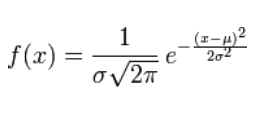那么，高斯分布的CDF为：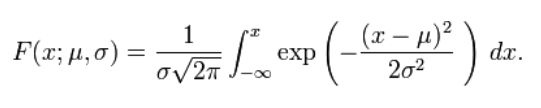而这个公式比较复杂，在写程序时，我们习惯用误差函数erf()来表示上面的CDF：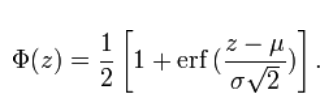其反函数称为反误差函数：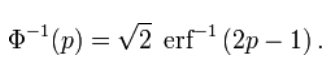2.方法二：Box-Muller方法
根据资料，我们可以先得到服从均匀分布的随机数再将服从均匀分布的随机数转变为服从正态分布。
其方法归纳为：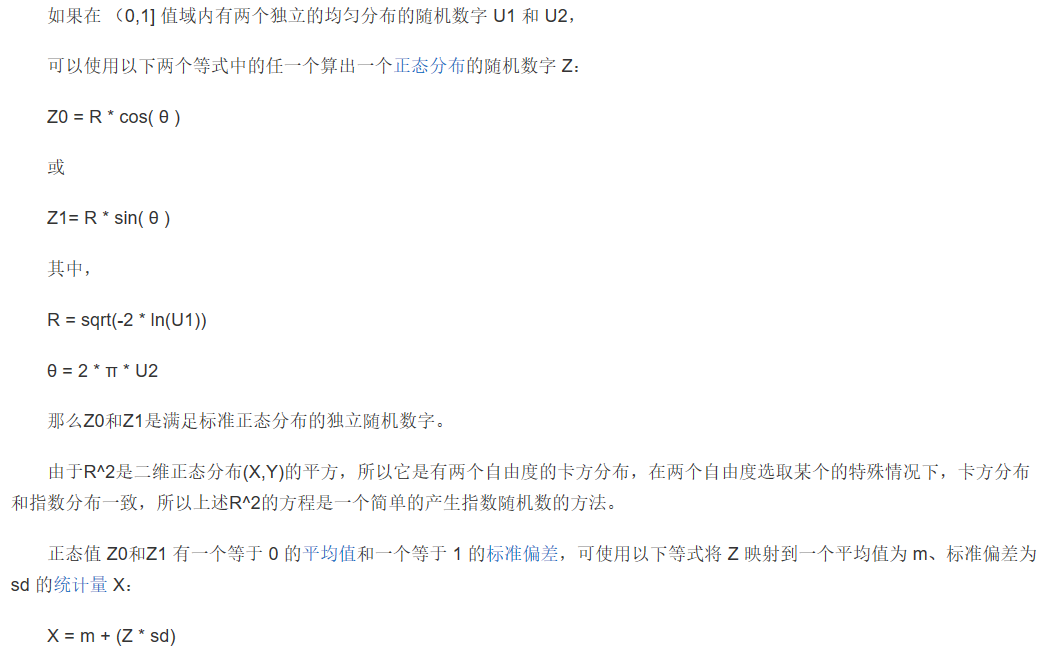下面是我程序设计的流程图

1.反函数方法的：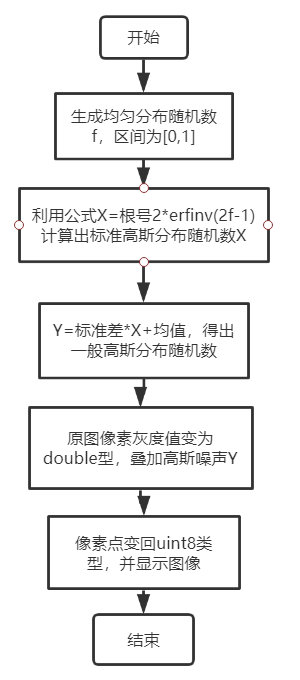2.Box-Muller方法的：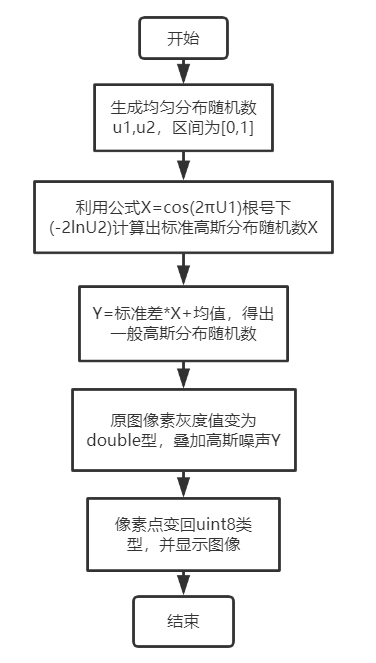下面是我的Matlab程序

clear;clc;
%% 原图像输入与显示
[width,height,z]=size(image);  %读取图像的大小
subplot(1,4,1);      %画子图个数，一共4个
imshow(image);      %画出原图
title('原图');      %标题
%% 高斯分布的均值与标准差赋值
mu=0;  %均值
sigma=0.1;  %标准差
%% Box-Muller方法产生高斯分布
u1=rand(width,height);  %生成图像大小的均匀(0,1)分布的u1，u2
u2=rand(width,height);
x=sigma*sqrt(-2*log(u1)).*cos(2*pi*u2)+mu;  %box-muller方法的公式书写，生成的是标准正态分布，再乘上标准差，加上均值，为最终的高斯分布
result1=double(image)/255+x;  %将图像变为double型，且数值区间在(0,1),再加上高斯分布形成的噪声
result1=uint8(255*result1);   %将double类型的灰度值变回为图像
subplot(1,4,2);
imshow(result1);
title('BM方法加高斯噪声后的图像');
%% 反函数方法
f=rand(width,height);   %生成(0,1)均匀分布的随机数
y=sqrt(2)*erfinv(2*f-1); %反函数方法，用反误差函数求，求出的是标准正态分布的随机数
y=sigma*y+mu;   %乘以标准差，加上均值，成为一般的正态分布随机数，作为高斯噪声
result2=double(image)/255+y;
result2=uint8(255*result2);
subplot(1,4,3);
imshow(result2);
title('反函数方法加高斯噪声后的图像');
%% 自带imnoise函数产生高斯分布，作为以上方法的参考与对比
P1=imnoise(image,'gaussian',0.01); %方差为0.01，均值为0
subplot(1,4,4);
imshow(P1);
title('自带函数加高斯噪声后');

得到的效果，用了最为经典的Lena图：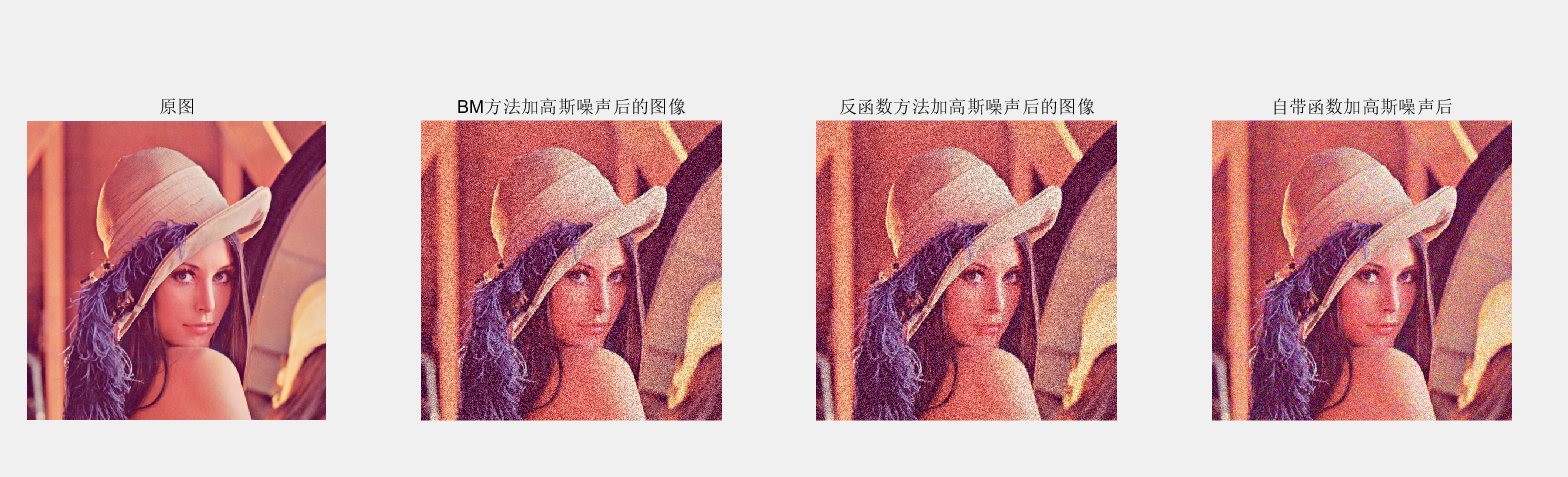展开全文• 本发明属于图像去噪技术领域，具体涉及一红外图像条状噪声消除方法。背景技术：红外成像系统能够捕获人眼无法看见的红外信息，在工业、医学、军事等领域具有重要的应用价值。红外焦平面阵列是当前红外成像系统使用...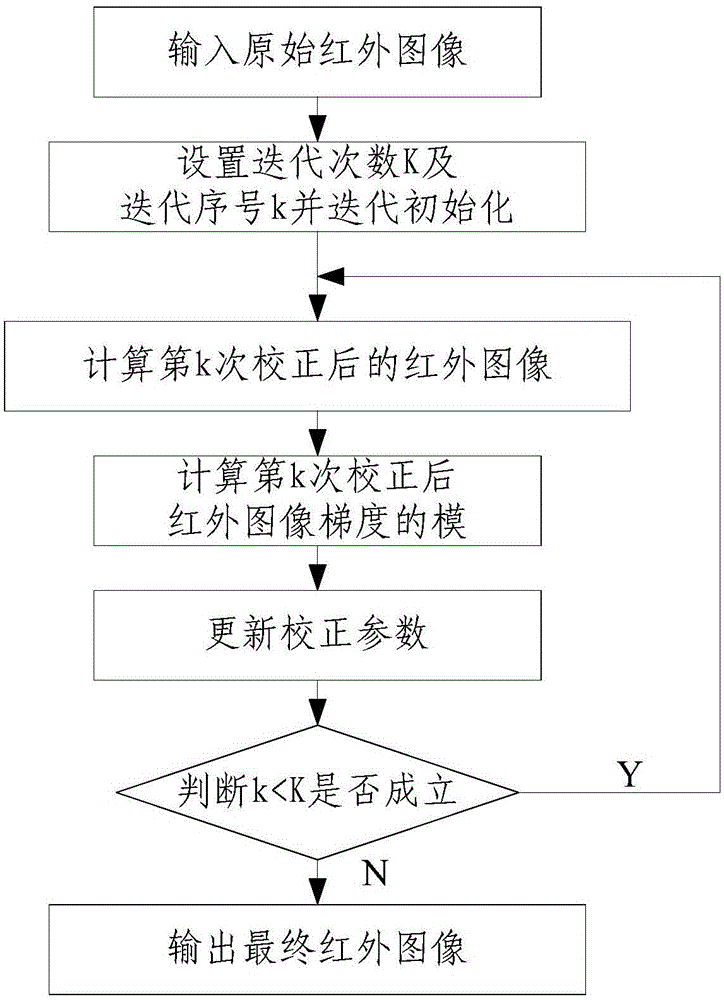本发明属于图像去噪技术领域，具体涉及一种红外图像条状噪声消除方法。

背景技术：

红外成像系统能够捕获人眼无法看见的红外信息，在工业、医学、军事等领域具有重要的应用价值。红外焦平面阵列是当前红外成像系统使用的主流技术，它具有体积小、制造成本低、敏感度高等优点。红外焦平面阵列通常采用列输出方式，即一个读出电路读取焦平面阵列中位于相同列的传感器响应。由于各读出电路无法做到完全相同，这种差异会在红外图像中产生明显的条状固定模式噪声。为了消除条状固定模式噪声，主要有两类方法：基于标定的固定模式噪声消除方法和基于场景的固定模式噪声消除方法。基于标定的噪声消除方法需要在拍摄过程中使用黑体(各处温度相同的物体)校正参数，因此会中断拍摄过程。基于场景的噪声消除方法仅使用连续拍摄的场景图像序列估计校正参数，但容易导致“伪影”现象。

上述两类方法均无法处理单幅含条状噪声的红外图像。传统的图像滤波方法无法有效消除条状噪声，同时还会导致图像模糊。Tendero和Gi l les提出使用Midway直方图均衡方法消除图像列间差异，达到消除条状噪声的目的，其优点是不需要使用待去噪图像以外的其它信息。但是这种方法计算量大，并且无法完全消除条状噪声。

技术实现要素：

本发明所要解决的技术问题在于针对上述现有技术中的不足，提供一种红外图像条状噪声消除方法，针对红外图像条状噪声的产生机理，通过最小化校正图像的能量估计校正参数，利用线性变换校正图像条状噪声，只利用红外图像本身就可以完成消除条状噪声，不需要额外的信息，在有效消除红外图像条状噪声的同时不会造成图像细节模糊，该方法计算量小，实时性好，便于推广使用。

为解决上述技术问题，本发明采用的技术方案是：一种红外图像条状噪声消除方法，其特征在于该方法包括以下步骤：

步骤一、输入原始红外图像：输入一幅带有条状噪声的原始红外图像Y(i,j)，原始红外图像Y(i,j)像素为M×N，其中，i＝1,2，…，M且j＝1,2，…，N；

步骤二、设置迭代次数K及迭代序号k并迭代初始化：设定迭代次数为K，同时初始化校正参数Gk(j)和Ok(j)，并给定初始迭代序号k＝0取G0(j)和O0(j)，其中，k≤K且K≥1，Gk(j)为第k次迭代后计算得到的红外图像第j列的增益校正参数，Ok(j)为第k次迭代后计算得到的红外图像第j列的偏置校正参数；

步骤三、根据公式Xk(i,j)＝Gk(j)Y(i,j)+Ok(j)，计算第k次校正后的红外图像Xk(i,j)；

步骤四、根据公式计算第k次校正后红外图像梯度的模|▽Xk|i,j，其中，|▽Xk|i,j为第k次校正后红外图像Xk(i,j)在(i，j)像素处的梯度的模值；

步骤五、根据公式更新校正参数Gk(j)和Ok(j)，其中，Gk+1(j)为校正参数Gk(j)更新一次后的增益校正参数，Ok+1(j)为校正参数Ok(j)更新一次后的偏置校正参数，Ek为第k次校正后红外图像Xk(i,j)的能量函数且λ为更新步长且为正常数；

步骤六、判断k＜K是否成立：当k＜K成立时，循环步骤三至步骤五；当k＜K不成立时，k取K，执行步骤七；

步骤七、输出最终红外图像XK(i,j)：根据公式XK(i,j)＝GK(j)Y(i,j)+OK(j)，计算输出第K次校正后最终红外图像XK(i,j)。

上述的一种红外图像条状噪声消除方法，其特征在于：步骤二中所述

上述的一种红外图像条状噪声消除方法，其特征在于：所述K取1～50000。

上述的一种红外图像条状噪声消除方法，其特征在于：所述λ取0～1。

上述的一种红外图像条状噪声消除方法，其特征在于：步骤五中为第k次校正后红外图像Xk(i,j)的能量函数对于其增益校正参数的偏导数且为第k次校正后红外图像Xk(i,j)的能量函数对于其偏置校正参数的偏导数且其中，|▽Xk|i+1,j为第k次校正后红外图像Xk(i,j)在(i+1，j)像素处梯度的模值，|▽Xk|i,j+1为第k次校正后红外图像Xk(i,j)在(i，j+1)像素处梯度的模值。

本发明与现有技术相比具有以下优点：

1、本发明引入增益校正参数和偏置校正参数揭示条状噪声的产生机理，通过最小化校正图像能量函数估计最优校正参数，图像的能量函数与图像梯度有关，图像梯度反映了噪声的强弱，最小化能量函数相当于尽可能地消除噪声。采用梯度下降法最小化能量函数，经过多次迭代得到最优校正参数，最后使用最优校正参数有效消除图像中的条状噪声，去噪效果明显。

2、本发明通过设置迭代次数，便于规定图像去噪所需时间，迭代次数设置有效，可靠稳定，使用效果好。

3、本发明只需要使用单幅红外图像就可以完成对图像的去噪，不需要测量其它额外的信息，步骤简单，计算量小，解决了红外图像中条状噪声问题，便于推广使用。

综上所述，本发明针对红外图像条状噪声的产生机理，通过最小化校正图像的能量函数估计校正参数，利用线性变换校正图像条状噪声，只利用红外图像本身就可以完成消除条状噪声，不需要额外的信息。在有效消除红外图像条状噪声的同时不会造成图像细节模糊，该方法计算量小，实时性好，便于推广使用。

下面通过附图和实施例，对本发明的技术方案做进一步的详细描述。

附图说明

图1为本发明采用的红外图像条状噪声消除设备的电路原理框图。

图2为本发明的方法流程框图。

图3为本发明未消除条状噪声的红外图像效果图。

图4为本发明消除条状噪声的红外图像效果图。

附图标记说明:

1—图像采集模块；2—图像处理模块。

具体实施方式

如图1和图2所示，本发明包括一种红外图像条状噪声消除方法，包括以下步骤：

步骤一、输入原始红外图像：输入一幅带有条状噪声的原始红外图像Y(i,j)，原始红外图像Y(i,j)像素为M×N，其中，i＝1,2，…，M且j＝1,2，…，N；

需要说明的是，像素为M×N的红外图像表示图像包含M行，每行有N个像素，本实施例中，采用图像采集模块1采集像素为288×384的红外图像并传输至图像处理模块2中处理，实际使用中，图像采集模块1包括红外焦平面探测器，图像处理模块2为计算机、高速微控制器或现场可编程门阵列，红外焦平面探测器的红外焦平面阵列通常采用列输出方式，即一个读出电路读取红外焦平面阵列中位于相同列的传感器响应，如图3所示，图像中包含了条状非均匀性噪声。

步骤二、设置迭代次数K及迭代序号k并迭代初始化：设定迭代次数为K，同时初始化校正参数Gk(j)和Ok(j)，并给定初始迭代序号k＝0取G0(j)和O0(j)，其中，k≤K且K≥1，Gk(j)为第k次迭代后计算得到的红外图像第j列的增益校正参数，Ok(j)为第k次迭代后计算得到的红外图像第j列的偏置校正参数，所述K取1～50000；

本实施例中，步骤二中所述

实际使用中，设置迭代次数K确保在指定的处理时间内，得到最优的增益校正参数和偏置校正参数，本实施例中，所述K取200。

采用增益校正参数和偏置校正参数对采集的图像信息校正，初始化增益校正参数和偏置校正参数，赋予保持图像初始状态下为图像采集模块1采集的原始图像。

步骤三、根据公式Xk(i,j)＝Gk(j)Y(i,j)+Ok(j)，计算第k次校正后的红外图像Xk(i,j)；

步骤四、根据公式计算第k次校正后红外图像梯度的模|▽Xk|i,j，其中，|▽Xk|i,j为第k次校正后红外图像Xk(i,j)在(i，j)像素处的梯度的模值；

步骤五、根据公式更新校正参数Gk(j)和Ok(j)，其中，Gk+1(j)为校正参数Gk(j)更新一次后的增益校正参数，Ok+1(j)为校正参数Ok(j)更新一次后的偏置校正参数，Ek为第k次校正后红外图像Xk(i,j)的能量函数且λ为更新步长且为正常数；

本实施例中，所述λ取0～1。

本实施例中，由于图像采集模块1采集的图像带有明显的条状噪声，需要调节合适的增益校正参数和偏置校正参数来消除条状噪声，为了使每次迭代过程中计算得到的新修正参数能达到更好的消除条状噪声的效果，采用梯度下降法更新校正参数，使得用新参数校正后的图像能量函数Ek更小，本实施例中，更新步长λ取0.0001，更新效果好。

实际操作中，λ取值不易过大，若λ取值过大，会造成算法无法收敛，迭代次数K的取值与λ的值有关，λ取的小，每一次更新量就小，需要更多的迭代次数使能量函数Ek最小化，K的取值就大。

本实施例中，步骤五中为第k次校正后红外图像Xk(i,j)的能量函数对于其增益校正参数的偏导数且为第k次校正后红外图像Xk(i,j)的能量函数对于其偏置校正参数的偏导数且其中，|▽Xk|i+1,j为第k次校正后红外图像Xk(i,j)在(i+1，j)像素处梯度的模值，|▽Xk|i,j+1为第k次校正后红外图像Xk(i,j)在(i，j+1)像素处梯度的模值。

实际计算时，更新校正参数Gk(j)和Ok(j)分别计算，能量函数Ek对增益校正参数Gk(j)的偏导数忽略偏置校正参数Ok(j)变化的影响，由于能量函数第k次校正后红外图像梯度的模且Xk(i,j)＝Gk(j)Y(i,j)+Ok(j)可得的值与Xk(i,j)和Y(i,j)的像素值均有关，能量函数Ek对偏置校正参数Ok(j)的偏导数忽略增益校正参数Gk(j)变化的影响，可得的值仅与本次迭代产生的红外图像Xk(i,j)的像素值有关，本实施例中，采用前向差分的方式求第k次校正后红外图像Xk(i,j)的能量函数对于其增益校正参数的偏导数以及第k次校正后红外图像Xk(i,j)的能量函数对于其偏置校正参数的偏导数，找到能量下降方向，实际计算时，能量函数Ek对增益校正参数Gk(j)的偏导数以及能量函数Ek对偏置校正参数Ok(j)的偏导数也可采用后向差分或中心差分的方式计算偏导数，找到能量下降方向，并且采用梯度下降法更新校正参数，处理速度快。

步骤六、判断k＜K是否成立：当k＜K成立时，循环步骤三至步骤五；当k＜K不成立时，k取K，执行步骤七；

步骤七、输出最终红外图像XK(i,j)：根据公式XK(i,j)＝GK(j)Y(i,j)+OK(j)，计算K次校正后最终红外图像XK(i,j)。

如图4所示，对于条状噪声去噪效果明显，实际操作中，根据图像处理时间与红外图像校正效果结合最优的条件下，本实施例中，在更新步长λ取0.0001下，经过200次迭代，输出最终红外图像XK(i,j)。

以上所述，仅是本发明的较佳实施例，并非对本发明作任何限制，凡是根据本发明技术实质对以上实施例所作的任何简单修改、变更以及等效结构变化，均仍属于本发明技术方案的保护范围内。

展开全文weixin_31235395 2021-02-10 04:05:58
• weixin_32121569 2021-04-18 09:36:29
• 转载：opencv-9-图像噪声以及评估指标 PSNR 与SSIM OpenCV 图形图像处理 图像噪声

LaoYuanPython 2021-04-05 19:51:26
• weixin_39675038 2020-12-19 13:45:15
• 图像滤波基础知识：图像与波的关系以及图像噪声知识 图像处理 滤波 图像噪声 计算机视觉 数字图像

LaoYuanPython 2021-04-05 20:55:47
• weixin_35927281 2021-01-14 04:06:21
• weixin_31095703 2021-04-18 06:46:04
• weixin_39789327 2020-12-19 13:45:14
• weixin_42062018 2021-04-11 20:50:13
• weixin_39610721 2020-12-19 13:46:13
• 给图像添加噪声（椒盐噪声，高斯噪声） opencv

qq_44805233 2021-10-12 15:51:01
• 【数字图像处理】全！使用MATLAB为图像添加噪声 图像添加噪声 matlab

ljh618625 2020-12-18 09:07:00
• CMOS图像传感器中的噪声来源分析 传感器 fpga 计算机视觉 图像处理 算法

qq_34901691 2021-06-14 00:30:11
• (学习笔记)图像噪声 计算机视觉 python 图像处理

weixin_39521013 2021-02-09 17:28:21
• 常见图像噪声及产生原因（高斯、泊松和椒盐噪声） 计算机视觉 机器学习

qq_28258885 2021-01-15 14:20:10
• 图像去噪-噪声模型 图像 噪声

Ango_ 2021-04-04 00:53:05
• （十九）图像噪声 python

m0_43609475 2021-02-08 10:56:20
• zyr_freedom 2021-03-17 15:44:48
• weixin_28295799 2021-04-18 09:52:44
• weixin_29661121 2021-04-22 18:21:12
• weixin_28937805 2021-04-20 04:40:17
• weixin_38485641 2020-12-29 22:12:45
• opencv-python数字图像处理学习4：对一副图像加噪声（高斯，椒盐等），进行平滑，锐化，观察其图像变化 opencv python 图像处理

qq_42112618 2021-10-16 14:45:07
• weixin_44935347 2021-07-04 10:19:59
• OpenCV——彩色图像添加椒盐噪声 opencv python c++

qq_36686437 2021-10-03 07:43:44
• weixin_39996798 2021-04-19 08:04:36...